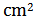Quantitative Aptitude Questions For SSC CGL Prelims 2018 | Set -2

Quantitative Aptitude Questions For SSC CGL 2018

Quantitative Aptitude Questions asked in SSC CGL Exam. Previous year Quantitative Aptitude Questions asked in SSC CGL. Welcome to the www.letsstudytogether.co online SSC CGL 2018 online preparation section. If you are preparing SSC CGL, CPO Exams 2018, you will come across a section on “Quantitative Aptitude “. Here we are providing you with “Important Quantitative Aptitude Questions For SSC CGL Prelims 2018”   based on the latest pattern of your daily practice.

This “Quantitative Aptitude Questions For SSC CGL 2018“ is also important for other SSC & Railway exams such as CPO, CHSL, RRB Group D, ALP & Technicians Exam 2018.

Quantitative Aptitude Questions For SSC CGL Prelims | Set -2

1.A monthly return railway ticket costs 25 percent more than a single ticket. A week’s extension can be done for the former by paying 5 percent of the monthly ticket’s cost. If the money paid for the monthly ticket (with extensions) is Rs. 84, then the price of the single ticket is?

A. Rs. 88

B. Rs. 30

C. Rs. 83

D. Rs. 64

D. Rs. 642. The ratio of number of students appearing for examination in 1998 in the states, A, B and C was 3 : 5 : 6. Next year if the no. of students’ in these states increases by 20%, 10% and 20% respectively, the ratio in states A and C would be 1 : 2. Find the no. of students who appeared for exam in the state A in 1998.

A. 3

B. 2

C. 5

Ratio of students in A & C in 1988 = 3 : 6 = 1 : 2
Increment in next year is same for the student
∴ The no. of students who appeared in the state A is 1998 are anyone of them =3, 6, 9, 12 etc.

3.Free notebooks were distributed equally among children of a class. The no. of note books each child got was 1/8 th of the no. of children. Had the no. of children been halved, each child would have got 16 notebooks. How many notebooks were distributed?

A. 256

B. 512

C. 128

D. None of these

D. 5124. A manufacturer undertakes to supply 2000 pieces of a particular component at Rs. 25 per piece. According to his estimates, even if 5% fail to pass the quality tests, he will make a profit of 25%. However as it turned out, 50% of the components were rejected. What is the loss of the manufacturer?

A. Rs. 13000

B. Rs. 12500

C. Rs. 15000

D. Rs. 14750

A. Rs. 130005.A cube has each edge 2 cm and a cuboid is 1 cm long, 2 cm wide and 3 cm high. The paint in a certain container is sufficient to paint an area equal to 54Which one of the following is correct ?

A. Both cube and cuboid can be painted

B. Only cube can be painted

C. Only cuboid can be painted

D. Neither cube nor cuboid can be painted

A. Both cube and cuboid can be painted6.The paint in certain container is sufficient to paint an area equal to 9.375 sq m. How many bricks of dimensions 22.5 cm × 10 cm × 7.5 cm can be painted out of this container?

A. 100

B. 200

C. 50

D. 175

A. 1007.Weight of a solid metallic sphere of radius 4 cm is 4 kg. The weight of a hollow sphere made with same metal, whose outer diameter is 16 cm and inner diameter is 12 cm, is

A. 20.5 kg

B. 15.5 kg

C. 16.5 kg

D. 18.5 kg

D. 18.5 kg8.Water flows at the rate of 10 m/min from a cylindrical pipe 5 mm in diameter. How long will it take to fill up a conical vessel whose diameter at the base is 40 cm and depth is 24 cm?

A. 51 min 12 s

B. 52 min 1 s

C. 48 min 15 s

D. 55 min

A. 51 min 12 s9. A well of inner diameter 14 m is dug to a depth of 15 m. Earth taken out of it has been evenly spread all around it to a width of 7 m to form an embankment. Find the height of embankment so formed.

A. 7 m

B. 5 m

C. 14 m

D. None of these

B. 5 m

Let Height of embankment be.10. The following pie chart shows the percentage distribution of the expenditure incurred in publishing a book. Study the pie chart and answer the following questions.
Expenditures (In percentage)If for a edition of a book, the cost of paper is Rs. 56250, then find the promotion cost for this edition ?

A. Rs. 21500

B. Rs. 22300

C. Rs. 22500

D. Rs. 22700

C. Rs. 22500

25% = 56250 then 10% = Rs.22500

Quantitative Aptitude E-Book for Banking, SSC and Insurance ExamsGet more stuff like this

Subscribe to our mailing list and get interesting stuff and updates to your email inbox.

LST Recommended : Oliveboard Mock Test Series